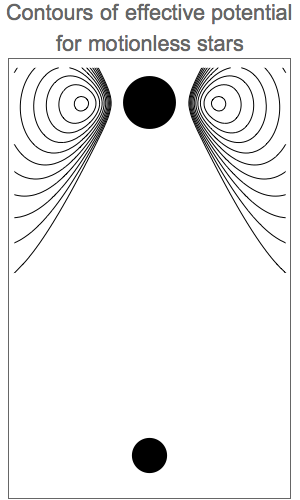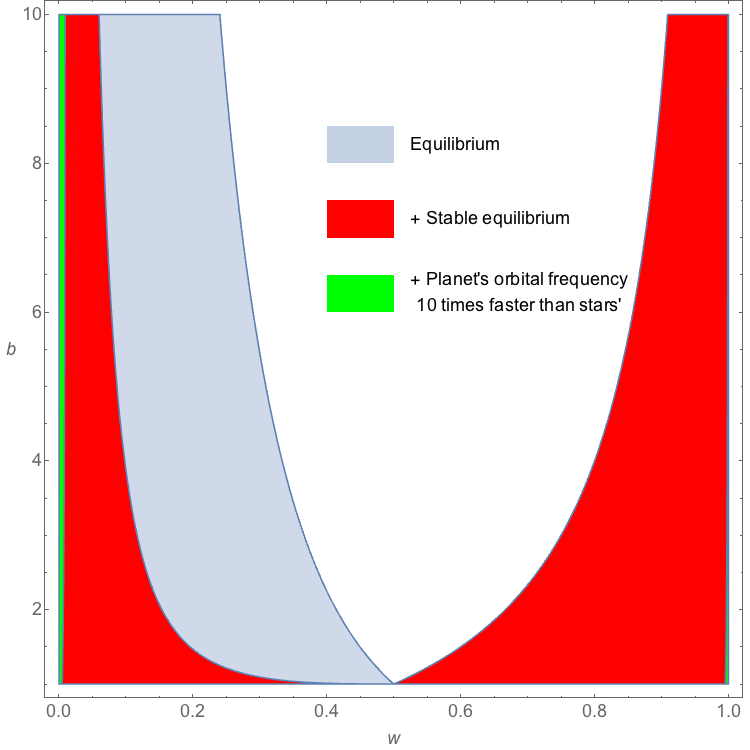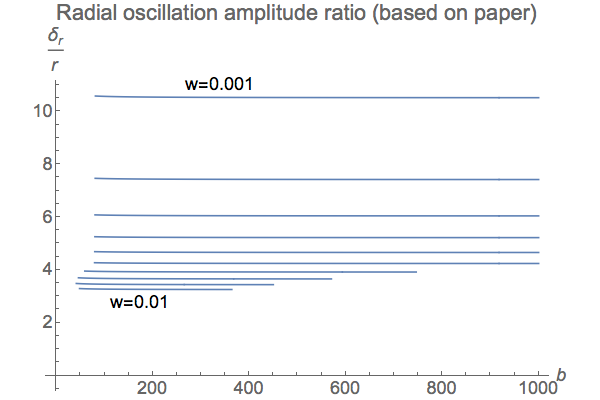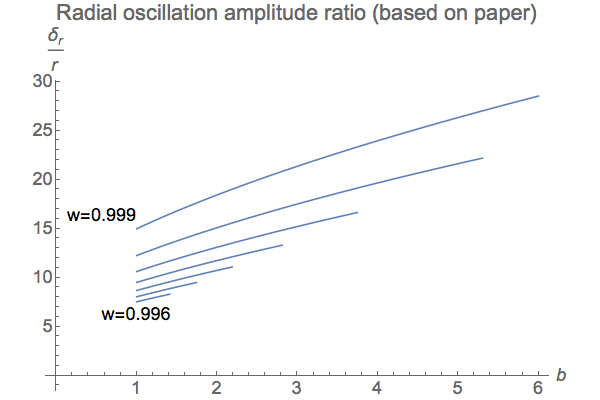# There Are No Corkscrew Orbits

## by Greg Egan

In May 2015, The Astrophysical Journal published a paper by E. Oks which stated:

... it is possible for OBSS [one-planet binary star systems] to have stable planetary orbits in the shape of a helix on a conical surface, whose axis of symmetry coincides with the interstellar axis ...

Take a moment to think about this extraordinary claim! A binary star system contains two stars orbiting their common centre of mass. The interstellar axis is a line joining those two stars. So, this axis will rotate. The paper is saying that a planet can move in a conical helix (a helix that wraps around a cone, rather than a cylinder), such that the axis of the cone remains aligned with the rotating interstellar axis!

The magazine New Scientist covered this with a breathless account that made it sound so exciting that I desperately wanted it to be true:

Even the craziest planets concocted by theorists still tend to trace conventionally near-circular orbits in a flat plane. Not so the corkscrew planet. Mind-bendingly, these worlds could exist in a sort of orbital limbo, spiralling about an axis between two stars in a binary system, pulled hither and thither by their competing gravities.

But wanting something to be true is not enough to make it so. If the two stars were motionless, it’s not hard to show that such “conic-helical” orbits really do exist. There is a certain amount of poetic licence in the language here: the orbits do not confine themselves precisely to a cone as a geometer would define it, but I have no objection to that. In fact, I have no problem with the entire early part of the paper which discusses the kind of stable planetary orbits that would exist, if the stars were somehow kept fixed.

However, when the author proceeds to examine how things change when the stars move in their orbit, he does not offer a convincing argument as to why the cone on which the planet’s orbit lies should rotate along with the stars. Other authors who have studied binary star systems with planetary orbits at large inclinations to the plane of the stars’ orbit report nothing of the kind. And both the numerical simulations I’ve performed, and some simple analytical calculations, strongly suggest that this is not the case. (A slightly more elaborate version of these calculations is given in a paper I’ve published.) I’ve also identified some specific problems with the paper.

[Update, 30 May 2016: On 20 May 2016, The Astrophysical Journal published an erratum, correcting some mistakes in the original paper: the missing factors of 2 and the radius of the planetary orbit that I noted below have now been inserted in some of the equations. Unfortunately, the corrections also introduce a brand new error, with the new version of equation (51) stating that the oscillations of the planetary motion occur entirely along the interstellar axis, with the radial oscillations being zero. This would turn the “conic-helical” orbits into “cylindrical-helical” orbits, if it were true, but it’s a mistake: a sign error in the change of coordinates from equation (48) to equation (50) has been fixed, while a sign error in the second line of equation (48), which was previously cancelling out that other error, now leads to an incorrect result in equations (50) and (51).

And none of these corrections address the most serious problem with the paper: the fact that the component of the planet’s angular momentum along the interstellar axis is not a conserved quantity as claimed.]

## Numerical Simulations

The numerical simulations are shown here.

## Analytical Calculations

It’s not hard to give an account of why the orbital plane of the planet will not remain orthogonal to the interstellar axis. A slightly more elaborate version of these calculations is given in a paper I’ve published in Celestial Mechanics and Dynamical Astronomy, “Polar Orbits Around Binary Stars”.

We will start by defining m(t) to be the projection of the planet’s angular momentum on the interstellar axis, measured in a rotating frame. Any torque that changes m(t) must be due to the centrifugal and/or Coriolis forces, because the gravitational forces from the stars will always apply torques perpendicular to the axis.

We will work in Cartesian coordinates fixed to the stars, with the x-axis as the interstellar axis, and the z-axis as the axis of rotation for the stars’ orbit.

The centrifugal force is F1 = ω2 (x(t), y(t), 0). The component of the torque along the x-axis due to the centrifugal force is:

T1 = y(t) F1,zz(t) F1,y = –ω2 y(t) z(t)

The Coriolis force is F2 = 2 ω (y'(t), –x'(t), 0). The component of the torque along the x-axis due to the Coriolis force is:

T2 = y(t) F2,zz(t) F2,y = 2 ω z(t) x'(t)

Now, suppose we approximate the motion of the planet with a circular orbit of angular velocity f and radius ρ, centred on the point (x1, 0, 0), with the plane tilted away from orthogonality with the interstellar axis by an angle α(t). We then have:

x(t) = x1 + ρ sin(α(t)) cos(f t)
y(t) = ρ cos(α(t)) cos(f t)
z(t) = ρ sin(f t)
x'(t) = –f ρ sin(α(t)) sin(f t)
T1 = –ω2 y(t) z(t) = –ρ2 ω2 cos(α(t)) sin(f t) cos(f t)
T2 = 2 ω z(t) x'(t) = –2 f ρ2 ω sin(α(t)) sin(f t)2

The component of the torque due to the centrifugal force, T1, will average to zero over one planetary orbit, because:

<sin(f t) cos(f t)> = <(1/2) sin(2 f t)> = 0

But the component of the torque due to the Coriolis force, T2, will not average to zero, because:

<sin(f t)2> = <(1/2)(1 – cos(2 f t))> = 1/2

So <T1> = 0, but <T2> = –f ρ2 ω sin(α(t)). So if the orbit is tilted even slightly, T2 will result in a persistent torque that will act to reduce the projection of the angular momentum onto the interstellar axis:

<m'(t)> = <T2> = –f ρ2 ω sin(α(t))

Note that m(t) itself is given by:

m(t) = y(t) z'(t) – z(t) y'(t) = f ρ2 cos(α(t))

The solution for α(t) that is compatible with the torque we have computed is then:

α(t) = ω t
m(t) = f ρ2 cos(ω t)
m'(t) = –f ρ2 ω sin(ω t) = <T2>

So to a first approximation, m(t) will be a cosine with angular frequency ω.

What if the orbit is initially not tilted at all? Then although the average torque over a full planetary orbit will be zero, if we look at the average torque over one quarter-cycle, there will be a small, but non-zero torque, with the same sign as that derived above. So the initial tilt of zero will not persist.

## Problems with the paperThe paper starts out by describing an effective potential for a planet that is orbiting the interstellar axis between two motionless stars, including both the gravitational potential energy and the part of the kinetic energy associated with a fixed value for m, the projection of the planet’s angular momentum on the interstellar axis. This is a standard method for separating out the orbital motion, leaving only the motion in the other two directions to be influenced by the effective potential. The image on the right shows the contours for this potential in a plane through the axis, for two stars with a mass ratio of b = 3.4 and a planet with an angular momentum of m = 0.810145; there is an energy valley in which the planet can orbit, at a distance of 0.998 along the axis (where 1 is the full length of the axis).

This section of the paper contains no errors that I could find. The problems start when the author tries to use exactly the same effective potential to analyse the situation when the stars are allowed to move around in their orbit. But if m changes, the effective potential changes, and the original version can no longer be used to describe the planet’s motion.

Why would m not change? (I’ve already explained in the previous section why I believe that it would.) Perhaps there is an intuitive appeal to the idea that the planet is “trapped” in a valley of the effective potential, which is fixed relative to the stars. So, as the stars move, the valley moves with them, and the planet has to come along for the ride, with the extra forces it feels in the stars’ rotating frame merely perturbing it slightly, so that it moves back and forth within the valley. The trouble with that analysis is that the extra forces don’t just nudge the planet itself. Because the effective potential depends on m, when a force acts on the planet in a way that changes m, the whole energy landscape shifts.

In any case, the author never really makes an argument for the constancy of m. He requires the planet to complete its own orbit much more rapidly than the stars complete theirs, so that the change in the orientation of the interstellar axis is a relatively slow process compared to the planet’s motion, but that in itself guarantees nothing: a slow process can still have a large cumulative effect. He offers no calculations in relation to this, and no reference to any established theorem; he simply refers to the stars’ rotation as being adiabatic (that is, gradual) as if that settles the matter, and then proceeds to assume that m will remain at least approximately constant.

Having made this assumption, the author then models the effect of the Coriolis force on the planet as a driven two-dimensional harmonic oscillator about the equilibrium point (the valley in the effective potential for the motionless stars), and derives amplitudes for the size of the oscillations in each direction. Because m isn’t actually constant as the stars orbit, this whole analysis is pretty much irrelevant to the real physics of the planet’s trajectory, but we will follow through its implications and see where they lead.

For a start, the author makes two errors in this calculation, first omitting a factor of 2 in the Coriolis force in equation (44), and then omitting a factor of the planet’s orbital radius in equation (46). So his final formulas for the forced oscillations in equation (51) are missing both those factors.

[Update, 30 May 2016: On 20 May 2016, The Astrophysical Journal published an erratum which inserts the missing factors ... but then introduces a new error into the results by claiming that the radial oscillations of the planet have an amplitude of 0. Checking the algebra shows that equation (48) in the original paper has a sign error, which remains uncorrected in the erratum, but now leads to errors in equations (50) and (51). But it’s also obvious on purely physical grounds that the new equation (51) can’t be correct, because it amounts to saying that the response of a two-dimensional driven harmonic oscillator is collinear with the driving force — the Coriolis force, which is directed along the interstellar axis — even though the interstellar axis is not a principal axis of the oscillator.]The paper never offers a concrete example of an orbit for which the oscillations described by equation (51) are relatively small (a prerequisite for the whole analysis), but it does deal with some other considerations.

The plot on the left is for w, the distance of the orbital plane along the axis from the lighter star to the heavier, and b, the mass ratio of the stars. The blue, red and green regions show how the valid parameter space shrinks as three necessary restrictions are imposed in turn:

• blue: that an equilibrium exists in the effective potential
• red: that the equilibrium is a stable one (a valley in the potential)
• green: that the planet’s orbital frequency is at least 10 times faster than that of the stars.

So, these two green slivers in the parameter space show the possibilities so far. But what happens if we go on to consider, also, the need for the forced oscillations about the equilibrium to be small?The plots on the right show the ratio of the radial oscillations to the complete radius of the orbit, if we take the erroneous formula for them in equation (51) of the paper seriously. The two plots correspond to the two cases for the green sliver: either for very small values of w (orbits close to the lighter star), or values near 1 (orbits close to the heavier star).

This ratio never drops below about 3. In other words, equation (51) of the paper implies that, for the regions of the parameter space allowed on other grounds, the oscillations in the planet’s orbital radius are always at least 3 times larger than the equilibrium orbital radius.

To put it another way, if we require the radial oscillations to be smaller than the orbital radius, the region of the parameter space to which the paper’s analysis is claimed to be applicable, according to its own criteria, is the empty set.If we correct for the missing factors in the paper’s version of the oscillation amplitudes, the situation becomes farcical in a different way.

The plots on the left show that the correct value for the radial oscillations becomes reasonably small when the orbit is very close to the lighter star (w is small), and the heavier star is much heavier (b is large).

But in that region of parameter space, we might as well be talking about the Earth as the lighter object and the sun as the heavier one. What the analysis in the paper would then amount to is the claim that if we put a satellite into orbit from pole-to-pole around the Earth, the plane of the orbit would be locked into facing towards the sun throughout the year. We have more than enough experience of the actual orbits of such satellites to know that this is not the case.

## References

 “Stable Conic-Helical Orbits of Planets Around Binary Stars: Analytical Results” by E. Oks, The Astrophysical Journal, 804:106 (11pp), 2015 May 10. Online at IOP Web Site.

 “Worlds of Pure Imagination” by Adam Hadhazy, New Scientist No. 3039, 19 September 2015, pp 34–38.

 “The Kozai Mechanism and the Stability of Planetary Orbits in Binary Star Systems” by K.A. Innanen, J.Q. Zheng, S. Mikkola & M.J. Valtonen, The Astronomical Journal, Volume 113, No. 5, pp 1915–1919, May 1997. Full article available at Smithsonian Astrophysical Observatory/NASA Astrophysics Data System.

 “Polar Orbits Around Binary Stars” by Greg Egan, Celestial Mechanics and Dynamical Astronomy, (2018) 130:5. Online at Springer Web Site.

 “Erratum: Stable Conic-Helical Orbits of Planets Around Binary Stars: Analytical Results” by E. Oks, The Astrophysical Journal, 823:69 (1pp), 2016 May 20. Online at IOP Web Site.Science Notes / There Are No Corkscrew Orbits / created Wednesday, 14 October 2015 / revised Sunday, 31 December 2017